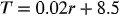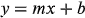1
32
views
10a
Problem

# Problem 10a

## Chapter 1: Functions and Models

###### Unlmited Homework Help for \$1 and win iPhone 13
Get a \$1 Homework+ and follow @oneclass.store on TikTok for a chance to win an iPhone 13.Textbook ExpertVerified Tutor
14 Oct 2021

#### Given information

Given the equation -where, T is temperature in "C" and r represents years since 1900.

#### Step-by-step explanation

Step 1.

In the equations likewhere m represents slope and b represents intercept. In the equation of temperature, 0.02 will be slope and 8.5 is the intercept.